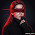## Monday, December 29, 2008

### Defining dynamic ranges / range names in Excel

Many time we use a range in our working which keeps on changing with addition/deletion of data. Common problem here is that user needs to keep refreshing / redefining the range.

However we can use dynamic range / range names and do away with redefining problem for ever. This is done with the help of OFFSET function of excel.

Offset returns a reference to a range that is a specified number of rows and columns from a cell or range of cells. The reference that is returned can be a single cell or a range of cells. You can specify the number of rows and the number of columns to be returned.
See the picture on defining dynamic date range name.Formula used is =OFFSET(Sheet1!\$B\$3,0,0,COUNTA(Sheet1!\$B:\$B),4)

See video for further explanation

Where all excel offset function can be used
You can use excel offset function wherever you use range in your formula. Such as Vlookup, Hlookup, Sum, Min, Max, Pivot tables etc.

Syntax
OFFSET(reference,rows,cols,height,width)
Reference is the reference from which you want to base the offset. Reference must be a reference to a cell or range of adjacent cells; otherwise, OFFSET returns the #VALUE! error value

Rows is the number of rows, up or down, that you want the upper-left cell to refer to. Using 5 as the rows argument specifies that the upper-left cell in the reference is five rows below reference. Rows can be positive (which means below the starting reference) or negative (which means above the starting reference).

Cols is the number of columns, to the left or right, that you want the upper-left cell of the result to refer to. Using 5 as the cols argument specifies that the upper-left cell in the reference is five columns to the right of reference. Cols can be positive (which means to the right of the starting reference) or negative (which means to the left of the starting reference).

If rows and cols offset reference over the edge of the worksheet, OFFSET returns the #REF! error value.

Height is the height, in number of rows, that you want the returned reference to be. Height must be a positive number.

Width is the width, in number of columns, that you want the returned reference to be. Width must be a positive number.

If height or width is omitted, it is assumed to be the same height or width as reference.

1.2.